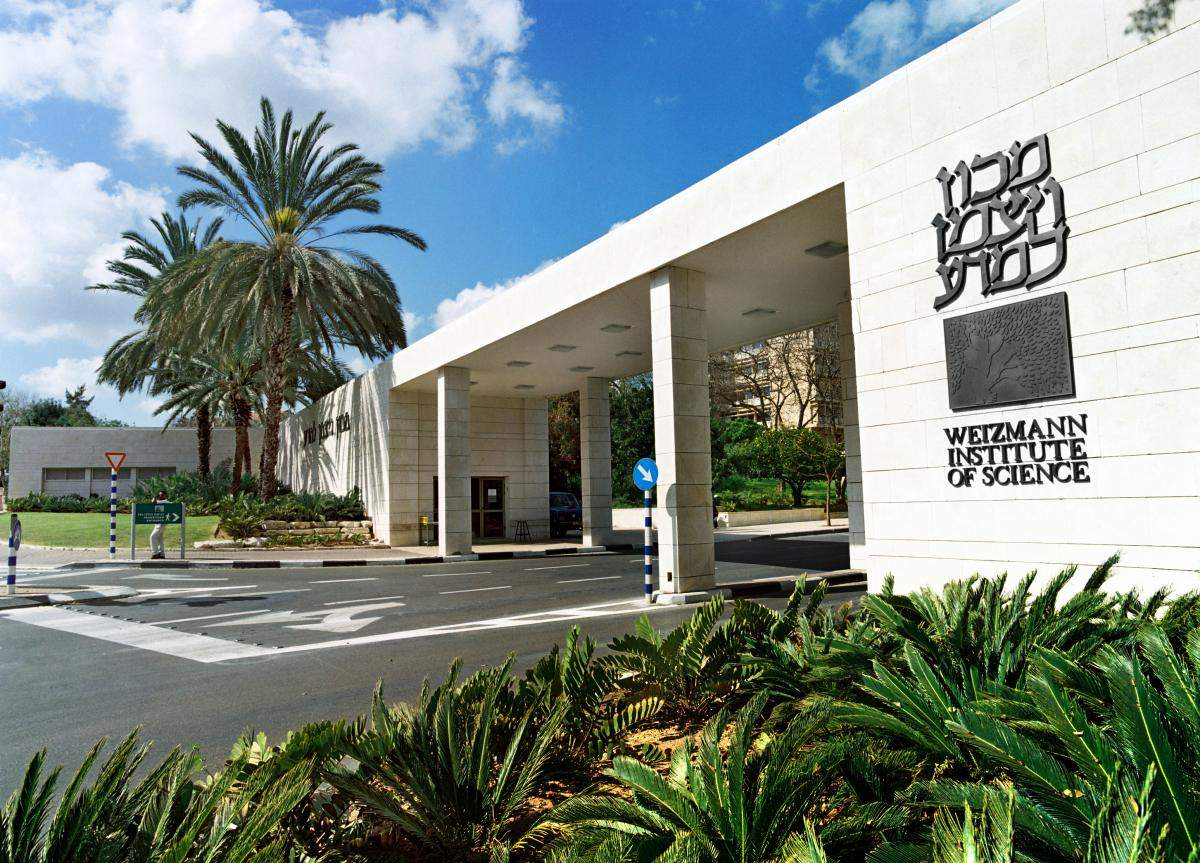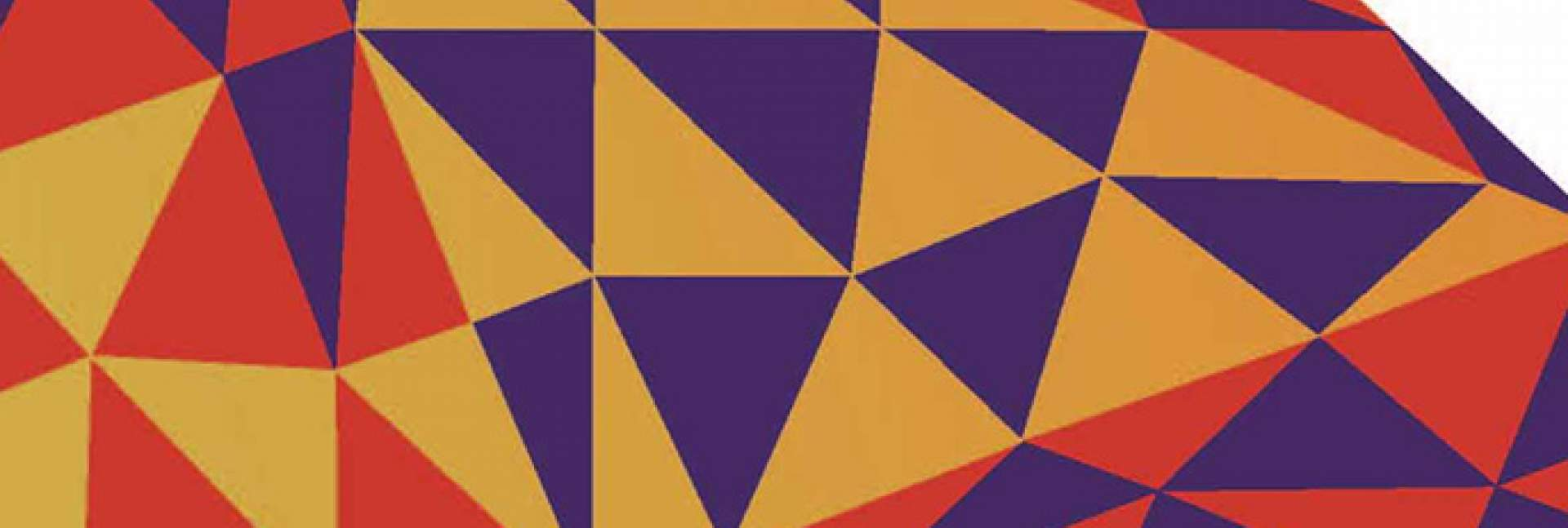# Bar Sagi Tiling: From Refrigerator Door to Mathematics Journal

## You are here

### Tags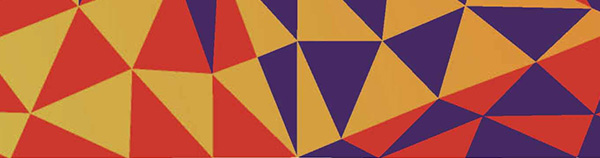Tiling has fascinated both mathematicians and artists since ancient times. The problem it poses can be described simply as revealing the ways to cover a plane using interlocking shapes and following a well-defined set of rules. Even in antiquity, the tiling of a plane using triangles, rectangles and regular hexagons was known. This long history of tiling might suggest there is nothing new to discover.  But mathematicians can surprise; indeed new tilings are discovered from time to time, most often by modifying the agreed-upon rules.

During the second half of the preceding century, two notable new tilings were discovered.  In the first, the tiles are chosen such that there are, necessarily, no repetitions in tiling the plane. This type is called aperiodic tiling, and it culminated in an important example that Roger Penrose presented at the end of the seventies.  Aperiodic tiling can be thought of as providing the inspiration for quasicrystals, which are aperiodic packings in three-space. Quasicrystals were first produced in the laboratory by Prof. Dan Schechtman of the Technion, and for this he was awarded the Nobel Prize in Chemistry in 2011.

Joseph showed that any such scaled-up triangle can be obtained by tiling with triangles from the original set

The second type is “self-similar tiling,” introduced by William Thurston in 1992.  In this type of tiling, starting from a given factor one must produce a finite set of shapes, any one of which can be enlarged by the given factor through joining members of the set.  More recently, Prof. Anthony Joseph of the Mathematics Department of the Weizmann Institute of Science has proposed “proper self-similar triangle tiling,” which he renamed “Bar Sagi tiling” in memory of his granddaughter who died at age fifteen of bone cancer.

Bar Sagi tiling is a generic name for tilings comprised of triangles derived from polygons.  First one fixes the number, call it n, of the sides of a polygon; this polygon is required to be regular (that is, it has equal angles and equal sides).  In such a polygon, a triangle may be inscribed by joining up the points of three successive vertices; if this is done in all possible ways, one gets the complete set of triangles based on n. If r,s and t are the number of sides of the polygon that can be counted between each of the three successive vertices, then r+s+t=n.  Two of the resultant triangles may be congruent: This is true if and only if their corresponding triples are the same up to permutation. However if r,s and t are all distinct (so that the triangle is scalene), then it is convenient to distinguish this triangle from its mirror image, as the one cannot be rotated into the other. In the diagram below, for example, for n=8 there are just five congruent triangles (four are shown in the octagon on the right and the fifth in the octagon on the left), two of which are scalene, making seven in total.  For n=10, eight triangles can be derived, four of them scalene, totaling twelve triangles.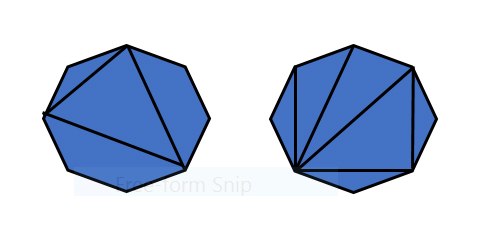The set of scale factors used in the tiling are the triangle side lengths relative to the shortest overall size length, taken to have length one.  Any one of the triangles in the set is chosen and then dilated by one of the scale factors.

Joseph showed that any such scaled-up triangle can be obtained by tiling with triangles from the original set. In this exercise there must, of course, be no gaps between the triangles; but more particularly, no corner of one triangle may break into the side of another. The latter requirement is an expression of the condition that the tiling be “proper.” He also showed that by imposing some natural extra conditions, the only sets of triangles that can exhibit self-similarity with dilation factors based on side lengths are those given using the above construction. This last condition on dilation factors means that the stacking of equilateral triangles, so common in the ancient world, is not a Bar Sagi tiling.

As in the case of Thurston self-similarity, this process can, in principle, be repeated.  However unlike Thurston tiling, the condition that the tiling be proper means that iterations are not immediately possible. Indeed, fulfilling this condition demands extra care in the way that tiling is implemented at each successive stage.

In the figure, one example of a Bar Sagi tiling is given.  It was obtained after three iterations, using the set of triangles obtained from a regular octagon.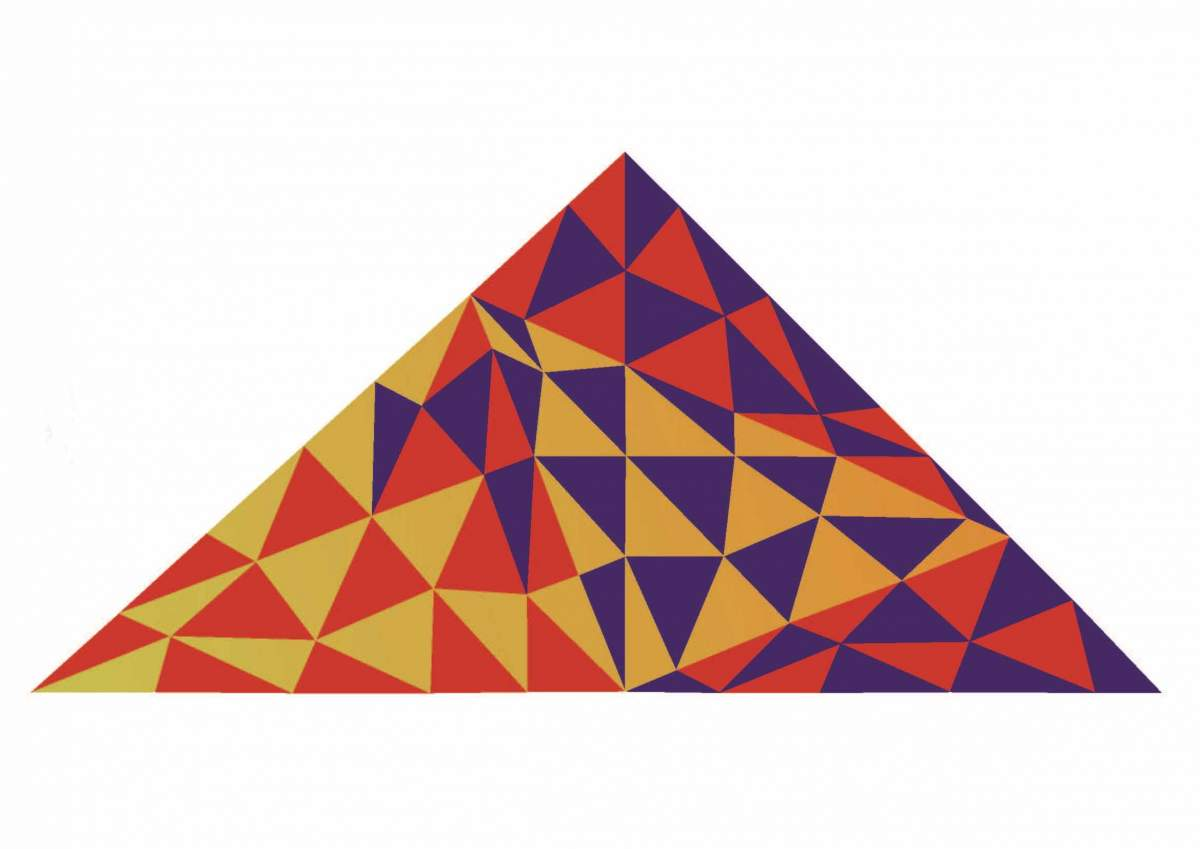In this somewhat evocative tiling, the colors are more than just decorative: According to a well-known theorem on coloring maps, proved by Kenneth Apel and Wolfgang Haken in 1976, four-coloring is sufficient for any map on a sphere (or a plane) and can also be necessary for certain maps. Here, in the first iteration of Bar Sagi tiling, two-coloring is possible ‒ that is to say, only two colors are needed so that no two triangles sharing a common edge have the same color. After a second or further iterations this may no longer be possible; indeed, in the above example at least three colors are needed. However, it is not yet proven that three colors are sufficient for Bar Sagi tiling.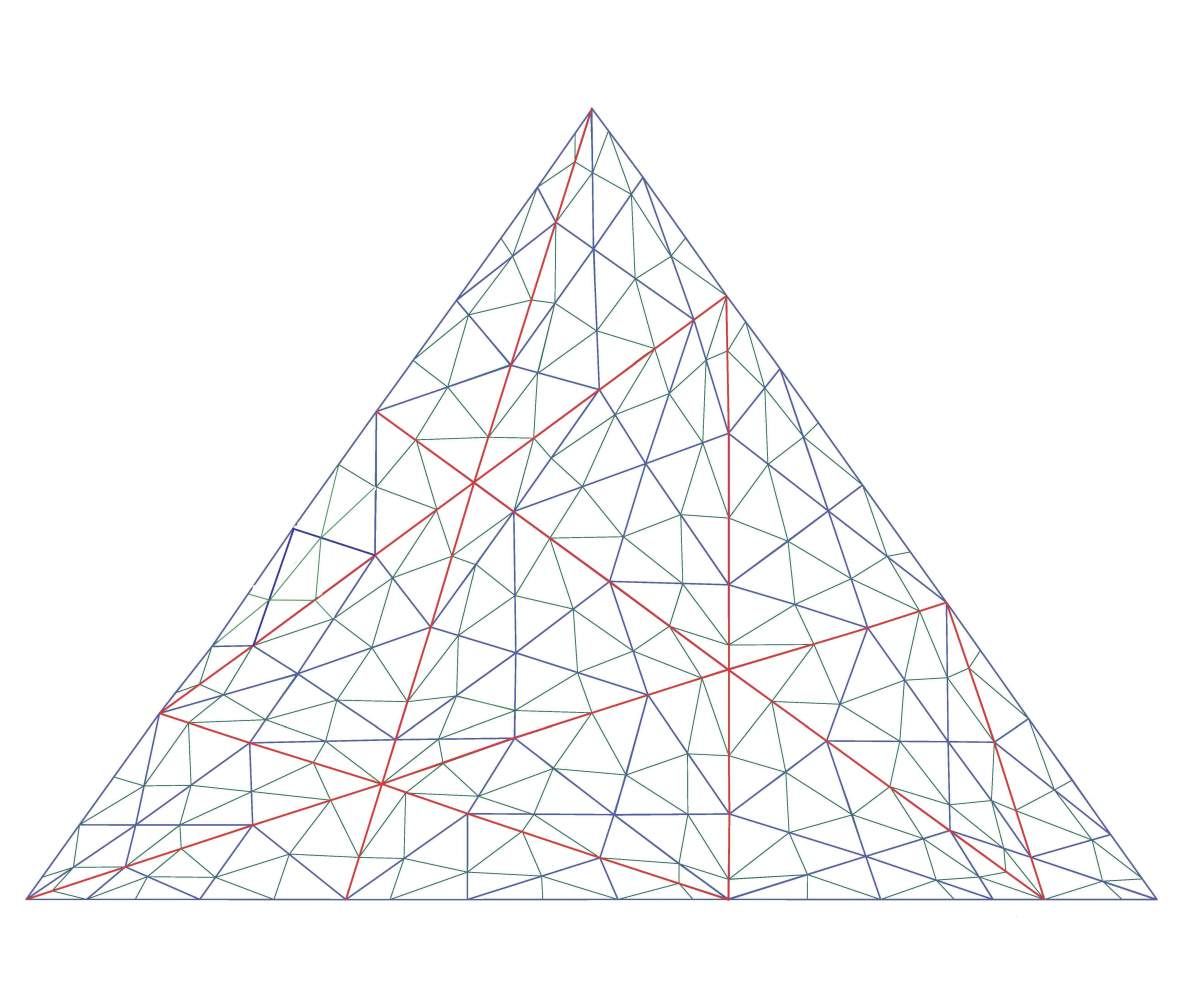One origin of the tiling considered here was a game Joseph had created for his granddaughter Bar (aged six at the time) by cutting triangles from colored paper with magnetic backing and letting her try to create tilings on the refrigerator door.

Another source of inspiration for the tiling was that the vertices of a proper self-similar triangle tiling can sometimes represent “weight diagrams” drawn in the plane. These diagrams are used in Lie algebra theory (Joseph’s main area of research) and can be projected to the plane in a rather specific manner. This presentation could not always be achieved, but the method led to the somewhat unexpected construction of certain “Weierstrass sections.”  These are important in separating the “orbits” that are obtained, for example, by rotating a sphere about its North-South axis.  In this context, such orbits are known as latitudes (or parallels).  Any (half) longitude going from the North to the South Pole cuts every latitude exactly once, thereby defining it.

The main thrust of Joseph’s work is in “non-commutative versions of algebraic geometry.”  A particular result of his has to do with the ways that classical and quantum mechanics, though similar, can exhibit calculable differences; and these can be thought to extend the well-known phenomenon of zero-point energy.Developing Bar Sagi tiling into a mathematical work dedicated to her memory was inspired by the beautiful poems written by Bar, most of which were found after her death. The poems relate in part to her painful and terrifying ordeal with bone cancer. They have been collected and published by Gvanim under the title “See Me Soar and Spread My Wings.”  Prof. Carlos Cardas, a leading oncologist at Cambridge University, UK, has declared this collection “to be mandatory reading for any aspiring cancer doctor.”

Now show me,

Prove to me you know!

What it means to be free!

What it means to fly!

Reach up and touch the sky

Let it out and let it start.

And maybe some day there'll be

A happy ending waiting for me

Full of love and friends

That's how I want my journey to end.

~ Bar Sagi

### Tags: